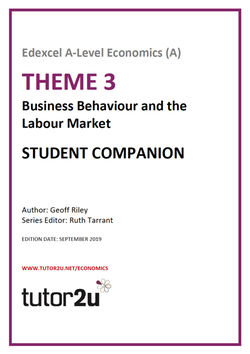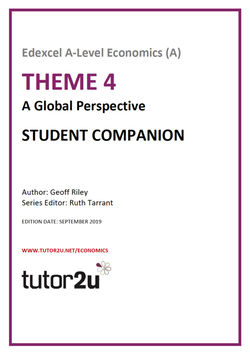�U�K�0������,�M<�\$����b�@�����m��e���vgɦ��mJ8�s �=���-bU�#b��5�')by�iDz�)�%������2.�&_NKpG�tJ|���Q�Gd��r:�>Fj0r��A� ؞�����F���&!��| �4`���,m�z�3��[��

# Economics

Explore EconomicsSearch
Study notes

# Explaining Fixed and Variable Costs of Production

• Levels: AS, A Level, IB
• Exam boards: AQA, Edexcel, OCR, IB

This study note provides a short introduction to fixed and variable costs for businesses in the short run

What is meant by the short run?

In the short run, at least one factor of production is fixed.

This means that output can be increased by adding more variable factors such as employing more workers and buying in more raw materials

What are fixed costs?

• Fixed costs do not change with output, firms must pay these even if they shut down
• Examples include the rental costs of buildings; the costs of leasing or purchasing capital equipment; the annual business rate charged by local authorities; the costs of employing full-time contracted salaried staff; the costs of meeting interest payments on loans; the depreciation of fixed capital (due solely to age) and also the costs of business insurance.
• Any business with significant capacity will have high fixed costs, for example a vehicle manufacturer that spends millions of pounds building a new factory and installing expensive and bulky capital equipment.

Total fixed costs (TFC)

Average fixed cost (AFC) = TFC / output

Average fixed costs must fall continuously as output increases because total fixed costs are being spread over a higher level of production.

A change in fixed costs has no effect on marginal costs. Marginal costs relate only to variable costs!

What are Variable Costs?

Variable costsvary directly with output – when output is zero, variable costs will be zero but as production increases, total variable costs will rise

Examples of variable costs include the costs of raw materials and components, packaging and distribution costs, the wages of part-time staff or employees paid by the hour, the costs of electricity and gas and the depreciation of capital inputs due to wear and tear

Average variable cost

(AVC) = total variable costs (TVC) /output (Q)

Total Cost (TC)

Total cost = fixed costs + variable costs

Average Total Cost (ATC or AC)

• Average total cost is the cost per unit produced
• Average total cost (ATC) = total cost (TC) / output (Q)

Marginal Cost

• Marginal cost is the change in total costs from increasing output by one extra unit
• The marginal cost of supplying extra units of output is linked with the marginal productivity of labour
• The law of diminishing returns implies that marginal cost will eventually rise as output increases
• At some point, rising marginal cost will lead to a rise in average total cost. This happens when the rise in AVC is greater than the fall in AFC as output (Q) increases

Calculating Costs – A Numerical Example

A numerical example of short run costs is shown in the table below. Fixed costs are assumed to be constant at £200. Variable costs increase as more output is produced.

 Output (Q) Total Fixed Costs (TFC) Total Variable Costs (TVC) Marginal Cost (the change in total cost from a one unit change in output) (TC= TFC + TVC) (AC = TC/Q) 0 200 0 200 50 200 100 300 6 2 100 200 180 400 4 2 150 200 230 450 3 1 200 200 260 460 2.3 0.2 250 200 280 465 1.86 0.1 300 200 290 480 1.6 0.3 350 200 325 525 1.5 0.9 400 200 400 600 1.5 1.5 450 200 610 810 1.8 4.2 500 200 750 1050 2.1 4.8

In our example, average cost per unit is minimised at a range of output - 350 and 400 units.

Thereafter, because the marginal cost of production exceeds the previous average, so average cost rises (for example the marginal cost of each extra unit between 450 and 500 is 4.8 and this increase in output has the effect of raising the cost per unit from 1.8 to 2.1).

An example of fixed and variable costs in equation format

If for example, the short-run total costs of a firm are given by the formula

SRTC = \$(10 000 + 5X2) where X is the level of output.

The firm’s total fixed costs are \$10,000

The firm’s average fixed costs are \$10,000 / X

If the level of output produced is 50 units, total costs will be \$10,000 + \$2,500 = \$12,500

Key Terms

Average fixed cost: Fixed cost per unit AFC= TC/Q

Average total cost: AC = cost per unit = TC/Q

Average variable cost: Variable cost per unit; AVC = TVC/Q

Diminishing marginal productivity: Falling MP as more units of a variable factor are added to a fixed factor

Long run production: Time period where all factor inputs are variable

Marginal cost: MC is change in total cost from supplying one extra unit

Short run production: Time period when at least one factor input is fixed

Sunk cost: A cost that cannot be recovered in a business closes down or leaves an industry

Total cost: TC = total fixed cost + total variable cost

Total fixed cost: Costs that do not depend on the level of output in the short run

Total variable cost: Variable costs are costs that vary directly with the level of output

#### Subscribe to email updates from tutor2u Economics

Join 1000s of fellow Economics teachers and students all getting the tutor2u Economics team's latest resources and support delivered fresh in their inbox every morning.

ASDAN, Home

New

• ### Turnover Rents

Student videos
• ### Marginal Cost Examples

Student videos
• ### Marginal Cost Explained

Student videos
• ### Contestable Markets (Online Lesson)

Online Lessons
• ### Short Run Costs - Law of Diminishing Returns (Online Lesson)

Online Lessons
• ### Barriers to Exit

Student videos
• ### Costs and Revenues - 60 Second Challenge (Knowledge Retrieval Activity)

Learning Activities
• ### Profits and Changes in Fixed Costs

Student videos
• ### Short run costs and revenues – finding profit maximisation

Student videos
• ### Short Run Marginal & Average Cost Explained

Student videos

### From the Blog

•### Coronavirus update: Rents – a heavy burden on firms as revenues shrink

31st July 2020

•### Dyson leaves the electric car market

11th October 2019

•### Free lunch? What if electricity was free?

26th January 2018

•### Sky abandoning the satellite dish - market contestability

25th January 2018

•### Byron in financial trouble seeks cuts in rents

9th January 2018

•### Contestable Markets - BC launches new Trans-Atlantic Low Cost Carrier

1st June 2017

•### Shut Down Point in Action: Jamie Oliver Closes 6 Restaurants

6th January 2017

•### The Economics of GAFA Businesses

5th December 2016

•### Netflix subscriber numbers soar - is 'video streaming' still a highly contestable market?

18th October 2016

•### Introduction to Teaching Contestable Markets (Webinar Resources)

7th October 2016

Study notes

Study notes

Study notes

Study notes

Study notes

Study notes

Study notes

Study notes

Study notes
• ### Positive consumption externalities

Study notes
• All Study notes ›

• 18

### Enrichment Tasks for A-Level Economics Students

Collections

•### Suggested Answers for the 2019 A-Level Economics Papers (AQA & Edexcel)

26th January 2020

• 37

Collections

• 22

### Head Start in A-Level Economics: the Transition Resource for Year 11 Students

Collections## Edexcel A-Level Economics Study Companion for Theme 3

• SKU: 02-4125-10991-01

• £30.00## Edexcel A-Level Economics Study Companion for Theme 4

• SKU: 02-4125-10992-01

• £30.00

### Teaching Vacancies

Tring School, Hertfordshire

• ### Teacher of Economics

Bishop Vesey’s Grammar School, Sutton Coldfield

New

ASDAN, Home

New
• ### Teacher of Business and/or Economics1 day left to apply

Hills Road Sixth Form College, Cambridge

• Browse all jobs ›

Recruitment